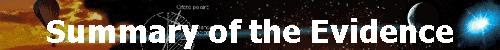Plasma Technology ] Theoretical Model ] [ Summary of the Evidence ] Synthesis Grid ] The Role of the Armed Forces ] Crop Circles ] Cattle Mutilations ] The Crime Weapon ] The Apparitions of Fatima ] The Crash of Flight TWA 800 ] The 1946 UFO Wave ] Roswell Crash Analysed ] A Colorado Case Study ] The Nullarbor Plain Case ]

###### u f o s :   t h e   m i l i t a r y   u n m a s k e d <!-- MSFPhover = (((navigator.appName == "Netscape") && (parseInt(navigator.appVersion) >= 3 )) || ((navigator.appName == "Microsoft Internet Explorer") && (parseInt(navigator.appVersion) >= 4 ))); function MSFPpreload(img) { var a=new Image(); a.src=img; return a; } // --> <!-- if(MSFPhover) { MSFPnav1n=MSFPpreload("_derived/back_cmp_ovnis010_back.gif"); MSFPnav1h=MSFPpreload("_derived/back_cmp_ovnis010_back_a.gif"); } // -->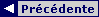<!-- if(MSFPhover) { MSFPnav2n=MSFPpreload("_derived/up_cmp_ovnis010_up.gif"); MSFPnav2h=MSFPpreload("_derived/up_cmp_ovnis010_up_a.gif"); } // -->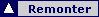<!-- if(MSFPhover) { MSFPnav3n=MSFPpreload("_derived/next_cmp_ovnis010_next.gif"); MSFPnav3h=MSFPpreload("_derived/next_cmp_ovnis010_next_a.gif"); } // -->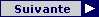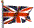Summary of the Evidence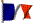Version française

Translation by George Hoskins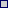#### Introduction

The idea is to examine the phase of the moon and its altitude on the horizon, as well as that of the sun, at the precise moment when a UFO phenomenon occurs, so as to expose a possible deception produced by a deliberate use of light effects.#### When the Moon is clear and near to the Horizon

When the moon is bright and is situated right beneath the horizon from the point at which a UFO phenomenon is observed, it produces a cone of black night of height h above the point O (see fig. 6-a). The luminous phenomenon, if produced within this cone of shadow, will benefit from the greater contrast and thus can be produced with less expenditure of energy. If one imagines here that this light phenomenon is fired from an aerial platform such as a dirigible balloon, for example, the ray of light produced will itself be within the clear night zone and thereby capable of being hidden by the brightness. Here we will speak of the Contrasting Effect due to the Moon, or CEM for short.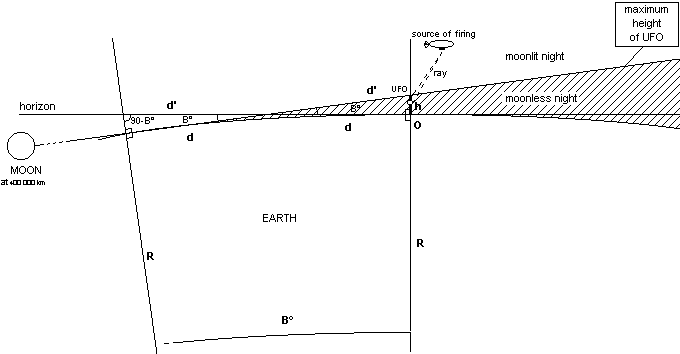Fig. 6-a

The height h of the cone of black night at the localisation point O of the UFO can be calculated as a function of the angle B traversed by the moon below the horizon and the radius R of the earth (6350 km):

h = (R/Cos (B-0.25°))-R   where   R = 6350 km

This formula gives the following values (for B below the horizon):

 B ~h (km) 1° 0.54 2° 2.96 3° 7.32 4° 13.62 5° 21.88 6° 32.11

As far as -4º below the horizon, the height >h of the shot fired is still reasonable (>13.62 km). We will therefore be able to speak of the CEM contrast effect if the moon is found between 0º and -4º beneath the horizon, but not beyond.

If the moon is above the horizon, the CEM contrast effect may still be seen up to +2º, for it is for this angle that the illumination on the ground becomes brighter than the illumination above ground, which puts an end to the CEM effect.

In order to demonstrate that the CEM contrast effect due to the moon is made use of by the instigators of UFOs, we will need to compare the percentage of times when it appears with the probability of its natural appearance.

In order to evaluate the natural probability of the CEM contrast effect at the moment of observation of the bright phenomenon, we can extrapolate the value of the angle B from the time t of the rising and setting of the moon. It is thought that the moon moves vertically on average 1º in 7 minutes when near the Earth’s horizon.

Within a 24-hour period the moon will twice cross the horizon in a pseudo-hazardous manner. In order to calculate the probability we can use, as being able to produce a CEM contrast effect, the slot of 42 minutes as follows:

-28 min ≤ t - t’ ≤ +14 min
which corresponds roughly to
-4° ≤ angle B ≤ 2°
where t = the time of the moon at 0º on the horizon and t’ = the time of observation,
when the moon sets and conversely when it rises

Natural probability of a CEM: 42 min x 2 = 84 min out of 24 hours, or about 1/17 or 5.8%. The natural probability of the CEM contrast effect is thus approximately 1/17 or 5.8%. We have been able to verify this calculation of the probability by carrying out a survey.#### When the Sun is near the Horizon

Like the moon, the sun produces a cone of shadow when it passes beneath the horizon of the observer (see fig. 7-a). This effect is much less sharp than that with the moon, however, since the strength of the sun’s brightness brings with it the gradual diffraction and diffusion of its light towards the ground. This is why it is only when the sun is -18º below the horizon that the "astronomical dusk" occurs, with the result that the light from the sun is definitively hidden from the observer.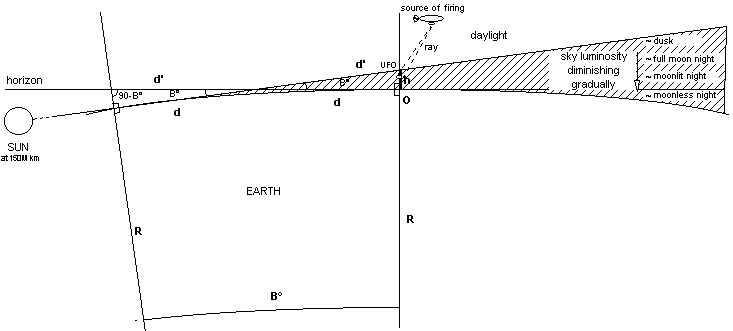Fig. 7-a

If the sun is beneath the horizon at the moment when a UFO phenomenon is seen, we will speak of a Contrast Effect due to the Sun, or CES for short.

The average duration of the night in France is considered to be about 12 hours, varying from a minimum of ~8 hours in summer to a maximum of ~16 hours in winter. For the calculation of the probability of a nocturnal CES we can use, as being able to produce a CES contrast effect, the slot of 126 minutes as follows:

-126 min ≤ t - t' ≤ +0 min
which corresponds roughly to
-18° ≤ angle B ≤ 0°
where t = the time of the sun at 0º on the horizon and t’ = the time of observation,
when the sun sets and conversely when it rises

Natural probability of a nocturnal CES: 126 min x 2 = 252 min out of 12 hours, or about 1/3 or 35%. We should note as an extreme case that during the last fortnight of June, when the angle B is above -18º for the whole night in the north of France, the natural probability of a CES is then 100% that night.

The natural probability of the nocturnal CES contrast effect is thus approximately 1/3 or 35%. We have been able to verify this calculation of the probability by carrying out a survey. NB: following a more thorough re-examination, this result is discussed in appendix E § 3.#### When both the Moon and the Sun are near the Horizon

It may be interesting to calculate the probability that the two effects, CEM and CES, described above, may combine during the night and thus increase their contrasting effect (see fig. 9-a). Let us remind ourselves that as the sun descends beneath the horizon, its influence on the luminosity of the sky diminishes to the point of resembling that of the full moon. We have used the angle of the sun at -8º below the horizon for our statistical study, although the balance between the brightness of the sun and that of the moon below the horizon appears to occur when the sun is actually much lower.

We will speak of a double contrast effect (CE2) when the CEM effect and the CES effect are present simultaneously and when the moon is opposite the sun in relation to the Earth (one chance in two), and is thus sufficiently illuminated to allow an effective CEM.Fig. 9-a

We can use, as being able to produce a CES contrast effect that could be combined with a CEM effect, the slot of 70 minutes as follows:

-126 min ≤ t - t' ≤ -56 min
which corresponds roughly to
-18° ≤ angle B ≤ -8°
where t = the time of the sun at 0º on the horizon and t’ = the time of observation,
when the sun sets and conversely when it rises

Natural probability of a CES that can be combined with a CEM: 70 min x 2 = 140 min out of 12 hours, or about 1/5 or 19.4%. Natural probability of a CE2: 1/17 x 1/5 x ½ = 1/170 or about 0.6%. The natural probability of a nocturnal CE2 contrast effect is thus approximately 1/170 or 0.6%. NB: following a more thorough re-examination, this result is discussed in appendix E § 3.#### Selection of Cases

The different UFO cases have been chosen from various sources which are mentioned for each group of cases. Note that all the cases given by the source have been examined so as not to bias our study.

We have studied the batches of cases which make up all the UFO waves from France published in the journal "Lumières dans la nuit" ("Lights in the Night") from No. 295 to No. 350, covering the period from January 1989 to December 1998, in order to try and ascertain whether there is some logic behind each of these waves. These cases are normally only reported if no explanation has been found for them and about two-thirds of them have multiple witnesses.#### Results

For the statistical analysis, the nocturnal cases are divided into two main categories according to whether the sky was clear or cloudy.

The result of the calculations is given below:

 Duration (min) Clear Sky Cloudy Sky CES CEM IM* (%) CE2 Accepted Cases CES CEM Accepted Cases OVERALL TOTAL OF CASES (excluding 5th Nov 1990) 113 27 8 266 5 0 16 (42.5%) (10.2%) (3.0%) (31.3%) (0%) Reminder of natural probabilities: 35% 5.8% 0.6% 35% 5.8% TOTAL SUM OF THE DURATIONS (excluding 5th Nov 1990) 1012.5 135.5 17 2147.5 113.5 0 878.5 (47.1%) (6.3%) (0.8%) (12.9%) (0%) OVERALL TOTAL OF CASES (including 5th Nov 1990) 159 67 43 318 5 0 16 (50.0%) (21.1%) (13.5%) (31.3%) (0%) Reminder of natural probabilities: 35% 5.8% 0.6% 35% 5.8% TOTAL SUM OF THE DURATIONS (including 5th Nov 1990) 1086 196 74 2224.5 113.5 0 878.5 (48.8%) (8.8%) (3.3%) (12.9%) (0%) AVERAGE OF IMs* DURING CEM EFFECTS Excluding 5th Nov 1990 75.2% Including 5th Nov 1990 85.8% Natural average 50%

*Illumination of the Moon

The results we obtained, without even including the great wave of 5th November 1990, which is very favourable towards our hypothesis, are significant and very different from the natural values which one would have expected to find. We noted 10.2% of CEM effects against 5.8% expected (1.75 times more than the natural value), 42.5% of CES effects against 35% expected (7.5% above the natural value) and 3% of CE2 effects against 0.6% expected (5 times more than the natural value). As regards the number of nocturnal cases when skies were cloudy, zero percent (0%) of CEM effects were obtained in contrast to 5.8%, and 31.3% of CES effects against 35% expected (thus 3.7% below the natural value), which might be explained by the the fact that these effects are useless in this context.

Nocturnal apparitions of UFOs seem to profit, therefore, more often than not (11.2% difference) from the CES effect when the sky is not covered, which seems symptomatic of the method used for their production, unless it were possible to prove that there exists a meteorological phenomenon whereby the sky covers itself more easily when the sun goes beyond -18º below the horizon.

The most striking results remain those linked to the movement of the moon since the latter is pseudo erratic and independent of human activities with a few rare exceptions. Normally it would have been necessary to study 1.360 cases (8 x 170) and not 266 in order to encounter 8 CE2 effects. This last result is the most remarkable.

We can evaluate the statistical value of the percentages obtained in the following manner:

 We have found 27 CEM cases out of 266 instead of 15.65 (266/17) which would have been the expected value. The probability of this fact being due to chance is about 1 in 625 (1.6‰), based on the following calculation: Value of the standard deviation SD: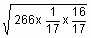That is, 3.84 And our deviation from the mean is 27-15.65 = 11.35 or 2.95 SD. Now, the probability of finding a deviation less than 2.95 SD is 0.9984 according to the standard normal distribution function table. The probability of finding a value greater than or equal to 2.95 SD is thus 1-0.9984 or 1.6‰ or 1 chance in 625.

 We have also found 8 CE2 cases out of 266 instead of 1.56 (266/170) which would have been the expected value. The probability of this fact being due to chance is about 1 in 10 millions, based on the following calculation: Value of the standard deviation SD: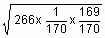That is, 1.25 And our deviation from the mean is 8-1.56 = 6.44 or 5.15 SD. Now, the probability of finding a deviation less than 5.15 SD is about 0.9999999 according to the standard normal distribution function table. The probability of finding a value greater than or equal to 5.15 SD is thus approximately 1-0.9999999 or 1 chance in 10 millions.

Looking back, we re-examined the 8 cases of CE2 effect and found that they all had multiple witnesses. We also re-examined the 27 cases of CEM effect and found that ~80% of them had multiple witnesses. Thus, for our statistics and very roughly speaking, if we had only made use of cases with multiple witnesses, approximately two out of every three, by arguing, for instance, in favour of their greater reliability, we would have obtained 8 CE2 cases out of 177, i.e. 4.5% instead of 3%, and 21 CEM cases out of 177, i.e. 11.9% instead of 10.2%, which would therefore have improved our results.

When one includes the great wave of 5th November 1990 in the calculation, the gap between natural probability and the actual results increases sharply. We get 21.1% of CEM effects against 5.8%, 50% of CES effects against 35%, and 13.5% of CE2 effects against 0.6% of natural probability.

These results suggest that the moon and probably also the sun are indeed in a particular configuration when bright UFO phenomena occur. It could be objected that if the correlation that we have researched turns out to be true, one may still question its real significance. If it is not a question of implementing a stratagem for creating luminous decoys, then we will have to find another explanation for the following fact:

 When a luminous UFO appears in the sky, it happens at least 5 times more frequently than it should that the sun is between -8º and -18º below the horizon, while the moon is between 2º and -4º on the opposite horizon.

We sincerely believe that it will be difficult to find an explanation other than that of the use of a luminous stratagem, because this anomaly is really very characteristic and is symptomatic of the fact that the sun and moon’s brightness would aptly complement each other in this configuration.

We have also examined the illumination of the moon when a CEM effect occurs. This illumination should normally be unpredictable and therefore have an average value of 50%. But the efficiency of the CEM effect is only guaranteed when the moon is rather bright, which supports our finding that the average illumination is actually 75.2% (as against 50%) and goes as high as 85.8% when we include in the calculation the wave of 5th November 1990. This result too is symptomatic of the method which is used. We can try to evaluate its statistical value by regrouping the 27 CEM cases according to the 4 bands of moon illumination for which we have calculated the natural probability. We obtain the following table:

 Illumination of the moon during the CEM effect 0 to 15% 15% to 50% 50% to 85% 85% to 100% 2% 13% 26% 45% 28% 79% 78% 69% 57% 56% 66% 97% 100% 95% 91% 91% 91% 91% 95% 96% 99% 95% 95% 95% 89% 98% 93% Distribution of the 27 values 2 3 6 16 Natural probability 25% 25% 25% 25%

The imbalance is already blatantly obvious in the table above, but its value can be calculated as follows:

 We have found 16 out of 27 CEM cases where the moon is illuminated to more than 85% instead of 6.75 (27/4) which would have been the expected value. The probability of this fact being due to chance is about 1 in 30,000, based on the following calculation: Value of the standard deviation SD: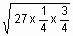That is, 2.25 And our deviation from the mean is 16-6.75 = 9.25 or 4.11 SD. Now, the probability of finding a deviation less than 4.11 SD is about 0.99997 according to the standard normal distribution function table. The probability of finding a value greater than or equal to 4.11 SD is thus approximately 1-0.99997 or about 1 chance in 30,000.

NB: a correction has later been made to these results in appendix E § 2 and § 3.